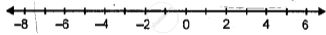# Fill in the Blank, Using the Following Number Line: an Integer, on the Given Number Line, is Greater than Every Number to Its_______ - Mathematics

Fill in the Blanks

Fill in the blank, using the following number line:An integer, on the given number line, is greater than every number to its_______

#### Solution

An integer, on the given number line, is greater than every number to its left.

Concept: Operation on of Whole Number on Number Line
Is there an error in this question or solution?

#### APPEARS IN

Selina Class 6 Mathematics
Chapter 7 Number Line
Exercise 7 (A) | Q 1.2